## 骨骼动画中的表情制作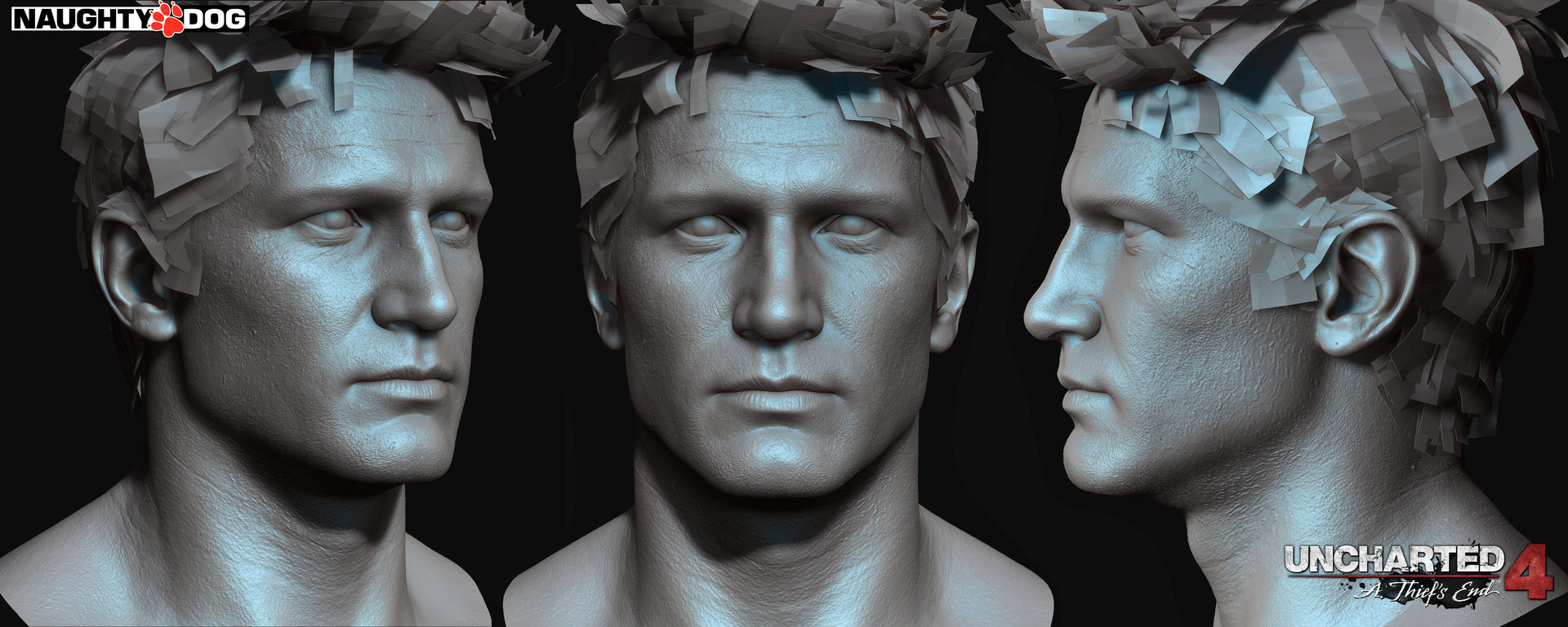1.SkinnedMeshRenderer在每帧计算骨骼数据的时候是否有做预计算优化？在抛开动作融合的需求上，实际上所有动画的骨骼计算结果都是可以离线计算的好的，运行时不需要再次从关节骨骼空间变换到模型空间，直接计算好模型空间的数据运行时输出即可。

2.为什么PlayerSetting 里的GPU Skinning选项永远都是*号？ 在Opengles3.0以及以下Es2.0 其寄存器数量不会超过256，大部分设备是224个顶点寄存器，假设我在这些设备上上传 1000个骨骼上去，Unity会成功进行GPU Skinning 还是fallback 到Cpu Skinning上？

3.使用Animator制作的大型状态机，如果带有大量动画，那么在加载的时候会加载整个状态机中包含的所有动画，此时加载性能会瞬间达到瓶颈，因此一般MMO项目都会自己来写额外分拆加载逻辑，而且Animator中的State融合时间又很难交给策划灵活配置，最后是很难做网络游戏的表现层和逻辑层分离，似乎是天生对单机游戏亲和力更好。

4.对Instancing的内置没有支持方案，以及没有做JobSystem的整合等等

5.存储空间和计算量大，由于Unity的骨骼使用Matrix4x4 矩阵，实际上骨骼计算使用3×4矩阵就可以满足， 不带缩放的骨骼甚至可以使用 8个float的双四元数就能解决，无论是在叉乘计算还是空间存储极限情况下性能会差距1倍左右

BlendShape方案

1.张嘴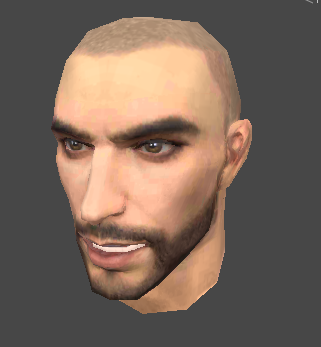2.闭嘴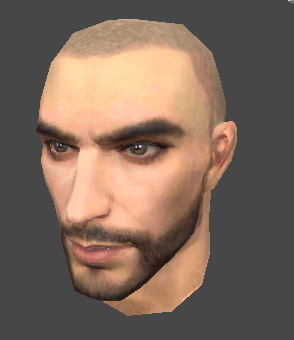3.闭眼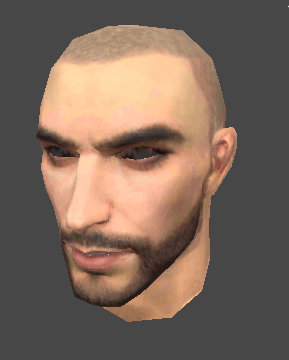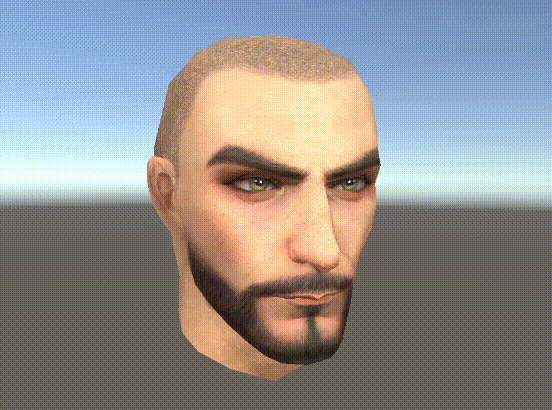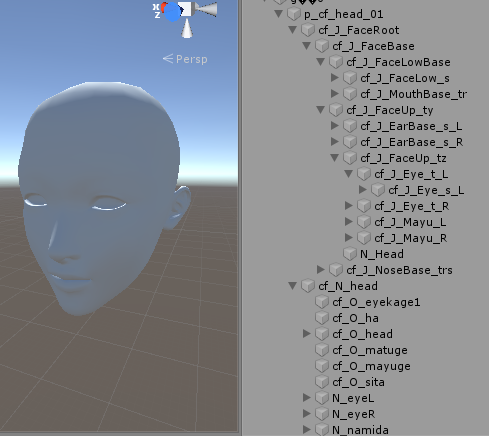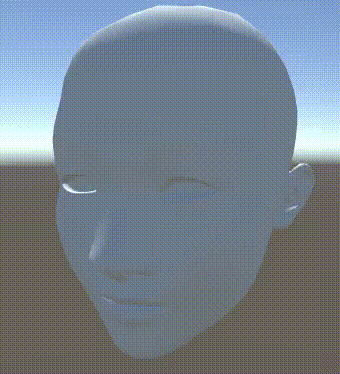123456789101112131415161718192021222324252627282930313233343536373839404142434445464748495051525354555657585960616263646566676869707172 void Start()     {         Mf = this.GetComponent();         Mr = this.GetComponent();         _baseVertices = BaseMesh.vertices;         _baseNormal = BaseMesh.normals;         _tmpVertices = new Vector3[_baseVertices.Length];         _tmpNormal = new Vector3[_baseNormal.Length];         _tmpMesh = new Mesh();         _tmpMesh.vertices = _baseVertices;         _tmpMesh.normals = _baseNormal;         _tmpMesh.triangles = BaseMesh.triangles;         _tmpMesh.uv = BaseMesh.uv;         _tmpMesh.MarkDynamic();         Mf.sharedMesh = _tmpMesh;         _vertexCount = BaseMesh.vertices.Length;         BlendShapeWeight = new float[BlendShapeMesh.Length];         _blendShapes = new BlendShape[BlendShapeMesh.Length];         for (int i = 0; i < BlendShapeMesh.Length; i++)         {             _blendShapes[i] = new BlendShape();             //差异顶点数量             List tmpBSVertexList = new List();             for(int j = 0; j < _vertexCount; j++)             {                 //变形器与基础顶点的差异                 if(_baseVertices[j] != BlendShapeMesh[i].vertices[j])                 {                     VertexDeltaData blendShapeVertex = new VertexDeltaData();                     blendShapeVertex.vertexIdx = j;                     blendShapeVertex.deltaVertex = BlendShapeMesh[i].vertices[j] - _baseVertices[j]; //delta position                     blendShapeVertex.deltaNormal = BlendShapeMesh[i].normals[j] - _baseNormal[j]; //delta normal                     tmpBSVertexList.Add(blendShapeVertex);                 }             }             _blendShapes[i].vertices = tmpBSVertexList.ToArray();         }         ModifyWeight();         _hasInited = true;     }     void ModifyWeight()     {         System.Array.Copy(_baseVertices, _tmpVertices, _baseVertices.Length);         System.Array.Copy(_baseNormal, _tmpNormal, _baseNormal.Length);         for(int i = 0; i < BlendShapeMesh.Length; i++)         {             BlendShape curBs = _blendShapes[i];             int deltaDataCount = curBs.vertices.Length;             for(int j = 0; j < deltaDataCount; j++)             {                 VertexDeltaData deltaData = curBs.vertices[j];                 int idx = deltaData.vertexIdx;                 _tmpVertices[idx] += deltaData.deltaVertex * BlendShapeWeight[i];                 _tmpNormal[idx] += deltaData.deltaNormal * BlendShapeWeight[i];             }         }         _tmpMesh.vertices = _tmpVertices;         _tmpMesh.normals = _tmpNormal;     }

btw:骨骼动画集大成的学习资料还是要靠隔壁国家的小黄油，推荐下最新的[AI少女]